# How can harmonic distortion be minimized

## Signal distortion and the harmonic distortion

If, after passing through an electronic assembly, the output signal is identical to the input signal except for a linear amplification factor, the signal processing is distortion-free. An ideal case that can almost never be achieved, since the signal in the transmission chain is influenced by capacitive, inductive and non-linear switching elements. Signal distortions are divided into linear and non-linear distortions and can be further divided, as shown in the reference-sensitive sketch.### Linear distortions

Electronic assemblies and circuits that have a linear voltage or current characteristic for each frequency cause linear distortion. They are referred to in English-speaking literature as linear distortion or frequency response designated. Ohmic resistances, capacitors and coils and the interconnection of similar components have linear behavior. At higher frequencies, linear distortions can be caused by the circuit structure, the board layout and the internal switching capacitance of the transistors and integrated circuits. The latter are often referred to as parasitic capacitances and cause frequency-dependent feedback.

In the case of linear distortions, only the amplitudes of the frequencies occurring in the input signal are changed in the output signal. Different frequency ranges can appear at the output with different transit times. The phase position of the input and output signals is then shifted to one another. No new frequencies appear in the output signal. The detection takes place with frequency analyzers by means of a signal analysis according to Fourier.

According to Fourier, every periodic signal can be represented from the fundamental and, if necessary, from the integer multiples, the harmonics. The fundamental oscillation, also known as the 1st harmonic, has the highest amplitude. The amplitudes of the harmonics decrease continuously towards higher frequencies. In the counting mode, the 2nd harmonic is thus the 1st harmonic, etc. The output signal has linear distortions if individual harmonics or entire frequency ranges of the input signal are influenced differently by linear switching elements.

### Amplitude distortion

The output signal of the two-port (quadrupole) to be examined shows a frequency-dependent amplitude response. Amplitude or attenuation distortion occurs. The following diagrams show examples of linear distortion for a low-pass and similarly dimensioned high-pass circuit. The input signal is a symmetrical square wave signal. The selected base frequency is 1 kHz. According to Fourier, the input signal contains all odd harmonics with decreasing amplitude.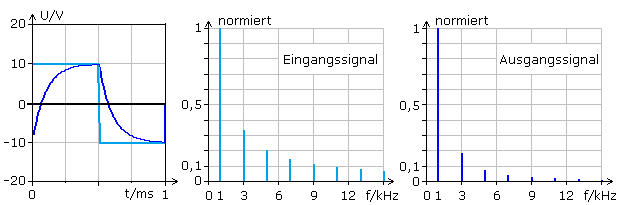In the timing diagram, the output signal (dark blue) is clearly distorted. The spectral analysis shows that the amplitudes of all higher harmonics are significantly lower compared to the input signal (light blue). The frequency range above the 5th harmonic is negligible. New frequencies do not appear in the spectrum. The input signal was linearly distorted by the low-pass filter.

The output signal after a high pass shows significantly higher amplitudes for the upper frequencies contained in the input signal. The timing diagram of the output signal is distorted completely differently. Since there are no new frequencies in the frequency spectrum, this is also linear distortion.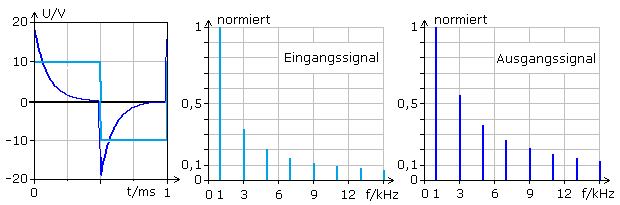Both examples show amplitude distortions which, depending on the area of ​​application, are desired and brought about in a targeted manner or are undesirable and thus disruptive. Influencing the sound in audio technology through equalizers or the simpler bass and treble adjustment using shelving filters uses low-pass, high-pass and band-pass circuits and creates the desired linear distortion. Audio amplifiers with bandwidths that are too low sound soft, the brilliance that comes from the high frequency ranges is missing. The harmonics above the audible range of 20 kHz also contribute to the audible sound quality.

If high-frequency or digital data signals are transmitted over long distances by cables, the low-pass nature of the cable results in linear distortions. This bandwidth limitation is annoying here because it makes clear signal detection at the output difficult or impossible.

### Time-of-flight distortions

Each input signal requires a certain, albeit very short time to reach the output of an electronic assembly. These transit times are dependent on the frequency and with the simultaneous oscillographic display of the input and output signals, they can be recognized as a phase shift. The delay distortions can therefore also be referred to as phase errors or phase distortion.

Phase errors occur particularly in active assemblies such as amplifiers. Amplifiers with a short signal rise time (slew rate) cause only slight delay time distortions within their working bandwidth. Regardless of this, the bandwidth-gain product, which represents a constant value, applies to each amplifier. For this reason, in the case of linear distortions, the amplitude and phase distortions cannot be completely separated from one another. As the gain increases, the usable bandwidth decreases, so that the high frequency components of the input signal occur with a lower amplitude in the output signal.

Almost all filter circuits produce phase shifts. In audio technology, frequency responses can be normalized using equalizers and existing amplitude errors in the listening area can be compensated for. The phase error will almost always increase. When dimensioning loudspeaker crossovers, knowledge of the frequency delays to the high, mid and low frequency chassis plays an important role in the final sound emitted.

### Nonlinear Distortions

Electronic assemblies with curved, non-linear characteristics cause non-linear distortion. Frequencies can always be detected in the output signal that are not present in the input signal. This effect can very well be intentional, because all modulation processes take place on curved characteristics and can thus be explained as nonlinear distortion.

One speaks of harmonic distortion when the new frequencies appear as integer multiples of the basic frequency. The amplitudes of the higher harmonics or harmonics usually decrease steadily with increasing frequency. In audio technology, non-linear distortions consisting of predominantly even harmonics are perceived as more pleasant. Their frequencies form an octave spacing from the fundamental. The first higher octave of a fundamental frequency has twice the frequency. Frequency patterns with integer multiples at octave intervals sound more pleasant to human ears. The aim is therefore to keep the amplitudes of the odd-numbered higher harmonics as low as possible.

Amplifier assemblies such as bipolar transistors, field effect transistors and electron tubes have differently curved control characteristics. Input signals with larger amplitudes are then no longer amplified symmetrically even with an optimally calculated operating point. The output signal is more distorted after a half-wave. Too large an input signal overdrives the amplifier, whereby the output signal is flattened and limited at both half-waves. In the distorted signal, Fourier frequency analysis can be used to detect new harmonics of the fundamental frequency.

Other assemblies with curved characteristics such as transformer transformers, tape heads and magnetic memories, diodes and VDR resistors also cause signal distortion. All of these distortions are called nonlinear distortion. The picture shows the harmonic components of an amplifier with a working point that is not optimally set.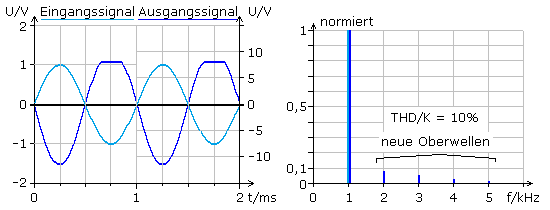The 1 kHz audio reference signal (light blue) is practically free of harmonics and consists of the fundamental frequency or 1st harmonic. The one-sided distorted output signal (dark blue) shows the newly added whole number higher harmonics in the spectrum on the right. The output signal shows non-linear distortions with a total amplitude component of 10%.

### The distortion factor or total harmonic distortion

Due to non-linear distortion and the occurrence of higher-frequency harmonics, a signal sounds shrill or clattering. Traditionally, non-linear distortions are specified using the distortion factor k. A similar parameter was later introduced as THD (total harmonic distortion). Both distortion factors represent a tense relationship.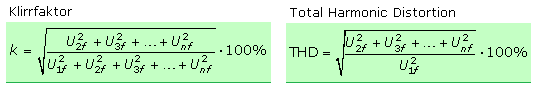The distortion factor is always less than one. The information is usually given in percent. The abbreviated equation presented above is sufficient for THD values ​​up to 5%. The distortion can also be specified as distortion attenuation or as a degree of distortion attenuation in dB, the value always being negative.A good transmission ratio is still given with a distortion factor of 5%. According to DIN 45500, hi-fi amplifiers may have a maximum of 1%, whereby even simple devices with factors <0.1% are better. The untrained hearing recognizes a distortion factor from 3% on the pure sound, with a little practice from 1%, while with a music or speech performance reliable recognition is only given from 7%.

The harmonic distortion depends on the size of the input voltage and on the frequency. Suitable negative feedback measures in the amplifier circuit minimize the distortion factor. The third harmonic is particularly pronounced in magnetic recording technology. The magnetization curve corresponds to a characteristic in the mathematical 3rd power, a cubic characteristic. They give preference to the third harmonic and thus the 4th harmonic. In these cases, the quality of the output signal is not judged by the distortion factor, but by a distortion coefficient. The cubic distortion coefficient or K3 factor of the magnetic sound recording is calculated using the following equation: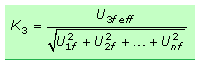If the distortion factor only arises from even harmonics, the signal sounds less distorted with the same distortion factor than for odd harmonics. Tone sequences at octave intervals, i.e. at double the frequency or an integral multiple of an octave frequency, are perceived by the ear as sounding more harmonious.

Harmonic distortions can be determined directly with a distortion factor measuring bridge. This device has one or more switchable sine wave generators with fixed measuring frequencies with negligibly small harmonic distortion factors. The sinusoidal signal is fed to the test object to be examined, for example an audio amplifier, as an input signal. Its output signal is analyzed by the distortion factor measuring bridge. In the measurement process, the frequency of the input signal is filtered out of the amplifier signal. The rms value of the residual signal is then the sum of all the new harmonic frequencies generated in the amplifier. The ratio of the residual signal to the total signal results in the distortion factor. Most of the measurements only take into account the first three to five harmonics corresponding to the 4th to 6th harmonics.

A frequency spectrum analyzer must be used for a very precise determination. This measuring device has a number of tunable, narrow-band resonant circuits of very high quality, which are in an integer frequency ratio. The resonance voltages are measured and evaluated for the total harmonic distortion THD / K.

### Nonharmonic Distortions

Most of the signals to be processed are a mixture of many different frequencies. When passing through amplifiers or other electronic assemblies, their non-linear characteristics can lead to amplitude modulation between individual signal frequencies. As a result, new frequencies appear in the output signal that are missing in the input signal. They are not in a harmonious relationship to one another and are perceived by the ear as disharmonies in audio signals and are perceived as particularly annoying. In relation to the circuit to be examined and the measurement method, non-harmonic distortions are referred to as difference tone distortions or intermodulation distortions.

### Difference tone distortion

The non-linearity of a narrow-band two-port leads to a high difference tone factor. Two different sine frequencies of the same amplitude, decoupled from one another, are used as the input signal. The rms voltages of the differential signals are measured at the output and set in relation to the rms value of the entire frequency mix. The second order difference tone factor d2 is of interest. It is caused on components with an asymmetrical parabolic characteristic. A symmetrical characteristic curve provides values ​​for the third-order difference tone factor d3. The smaller the frequency spacing of the input signals, the further the difference signals are shifted to low frequencies and the sum frequencies that also arise are outside the bandwidth of the two-port.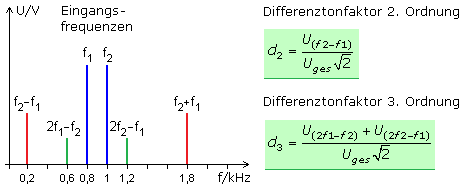### Intermodulation distortion

A special two-tone measurement method is used in the field of electroacoustics. According to the DIN 45500 Hi-Fi standard, the two sine frequencies 250 Hz and 8000 Hz are applied to the input of the test object with an amplitude ratio of 4: 1. For evaluation, the sum of the rms values ​​of the sum and difference frequencies that appear at the output is set as modulation products in relation to the rms value of the higher signal frequency. Here, too, mostly only second and third order intermodulation factors are taken into account. A general equation for calculating the intermodulation factor can be set up by inserting a distance factor q. The distance factor stands for the integer distance between the side frequencies in relation to the higher measuring frequency f2 that is being modulated.The calculation can be done either with the effective or peak values. The geometric addition is not necessary for the two simple equations above, since all signals have a fixed phase relationship with one another. The result is given in percent or decibels. With good audio amplifiers, the intermodulation factor is less than 0.5%.

### Mathematical background to IM

In the chapter on amplitude modulation on the diode characteristic it is shown that the non-linearity can be described mathematically by a power series. In addition to the square member, it also contains members of a higher order, through which further mixed products can arise. In the power series, the amplitudes decrease rapidly with increasing order. To determine the intermodulation distortion (IM), it is therefore sufficient to take into account the intermodulation products of the second IM2, at most the third order IM3.

### 2nd order intermodulation

Before the modulation, the two input signals are additively superimposed. With the sum signal, new frequencies arise at the non-linear transfer function, including the difference and sum frequencies as with amplitude modulation. The intermodulation corresponds mathematically to a multiplication of the frequencies.The intermodulation can be examined and compared with the mathematical result with a circuit set up in the laboratory or more simply with a simulation program on the PC. As input frequencies, 1 kHz and 10 kHz with the same amplitude are added linearly on a resistor network. The sum signal goes to both inputs of a full multiplier with a gain of 1. The oscillograms and Fourier analyzes of the signals are displayed.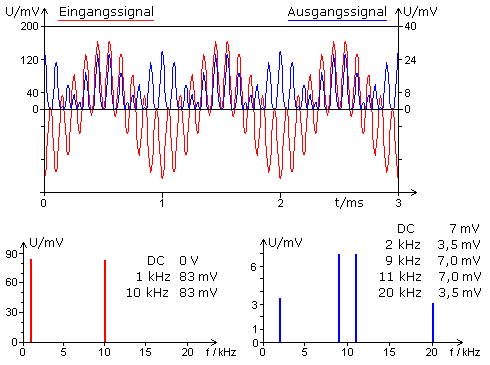The oscillogram of the input signal (red) has no DC voltage component and runs symmetrically to the zero line, while the output signal (blue) with its DC component lies above the zero axis. The values ​​determined by the Fourier analysis of the output signal correspond to the equation derived above. The new DC voltage component can shift the operating point of the circuit and thereby increase the intermodulation factor.

### 3rd order intermodulation

The two additively superimposed input signals are distorted by a 3rd degree characteristic curve. Mathematically, the third power is to be calculated, a process with a lot of written calculation effort.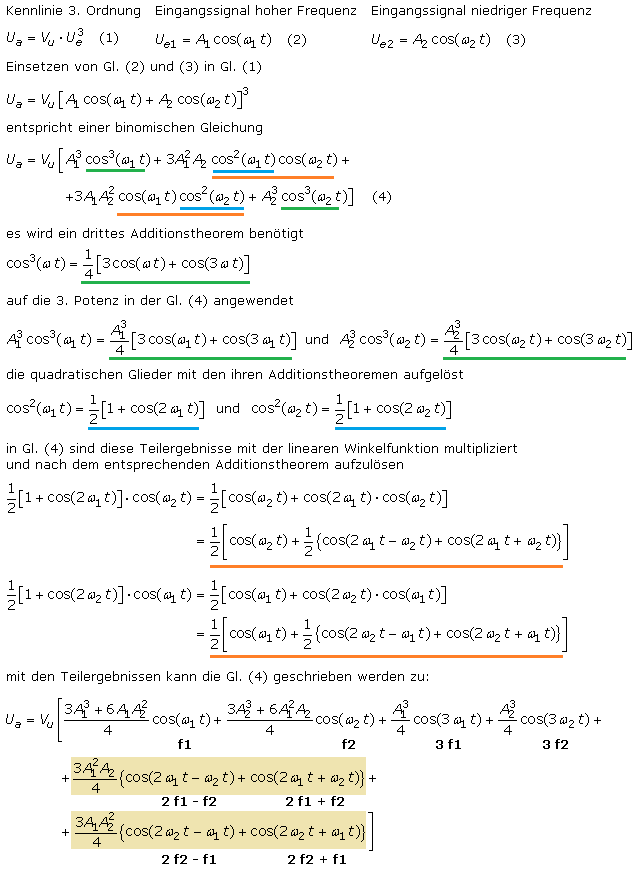The two colored expressions of the final equation stand for intermodulation products. The cosine is a symmetrical function and cos (x) = cos (−x) applies. Applied to cos (2 · f2 - f1) we get cos (f1 - 2 · f2). With cos (f1 + 2 · f2), this forms the value pair in the numerator of the equation that determines the intermodulation factor.

The input frequencies 1 kHz and 10 kHz generate additional frequencies of 3 kHz, 30 kHz, 8 kHz, 12 kHz, 19 kHz and 21 kHz on a 3rd order (cubic) characteristic. The spectral distribution with its amplitude values ​​could be verified with simulation software. Two sinusoidal signals with 1 kHz and 10 kHz were added linearly and fed as an input signal to a multiplier. The output signal and the addition signal form the input signals of the second multiplier. Its output signal corresponds to the third power of the input signal. The picture shows the Fourier analysis with the measured amplitude values. They agree with the gain factors of the equation derived above.### Jean-Baptiste Joseph Baron de Fourier ✖

The French mathematician and physicist Fourier (1768-1830) received his training in the monastery of Saint-Benoît-sur-Loire. He was actively involved in the French Revolution. As a research assistant on the Napoléon Bonaparte expedition in Egypt, Fourier published studies on Egyptian antiquity. He was appointed baron in 1808.

Fourier made a name for himself through his mathematical and physical work. He developed the analytical theory of heat propagation and heat conduction using trigonometric series and the corresponding integrals. In his honor they are called Fourier series today.Many scientific processes can be described using the methods of Fourier analysis and Fourier synthesis. He also introduced the concept of the physical dimension.